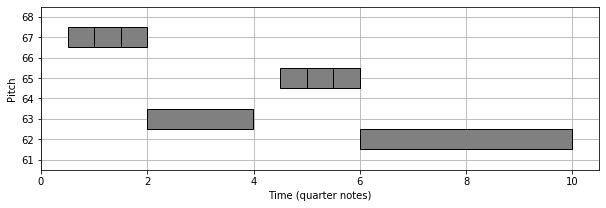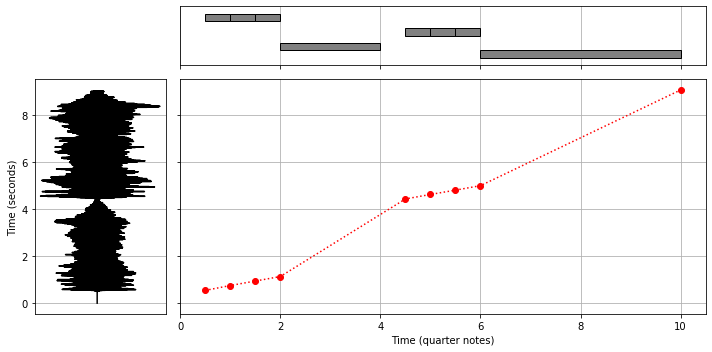# MTD: A Multimodal Dataset of Musical Themes for MIR Research¶

This Jupyter notebook accompanies the Musical Theme Dataset (MTD) and demonstrates how to use it. The dataset is described in the following paper.

Frank Zalkow, Stefan Balke, Vlora Arifi-Müller, and Meinard Müller
MTD: A Multimodal Dataset of Musical Themes for MIR Research
Transactions of the International Society for Music Information Retrieval (TISMIR), 2020, under review.

The following website accompanies the paper and presents all links for accessing the MTD.
https://www.audiolabs-erlangen.de/resources/MIR/MTD

This notebook assumes that you are familiar with Python for music processing. In particular, we use Python and Jupyter with standard packages like pandas, pretty_midi, librosa, and matplotlib. If you want to familiarize yourself with Python for music processing, we recommend visiting the Python Notebooks for Fundamentals of Music Processing.

In the first code cell, we import some Python packages.

In :
import os
import glob
import json

import IPython.display as ipd
import numpy as np
import pandas as pd
from pretty_midi import pretty_midi
import librosa
import librosa.display
from matplotlib import pyplot as plt
from matplotlib import patches
%matplotlib inline


## Files¶

We now start by specifying an identifier for a musical theme. As an example, we define the identifier for the first theme of Beethoven's Fifth Symphony. Alternatively, you may remove the comments below to get a random id from the dataset. If you like to search for a specific theme identifier, you can use the MTD overview website.

In :
# specify identifier
mtd_id = '1066'

# or get random identifier
# files = glob.glob(os.path.join('MTD', 'data_EDM-corr_MID', '*.mid'))
# mtd_id = os.path.basename(np.random.choice(files)).split('_')[3:]

print(mtd_id)

1066


The following code cell defines all file paths needed for the notebook. All paths are based on the MTD identifier and are printed at the end. This notebook will describe the files and show how to read their content.

In :
def get_file(fn):
files = glob.glob(fn)
assert len(files) == 1, '{} does not exist.'.format(fn)
return files

fn_corr_mid = get_file(os.path.join('MTD', 'data_EDM-corr_MID', f'MTD{mtd_id}_*.mid'))
fn_corr_csv = get_file(os.path.join('MTD', 'data_EDM-corr_CSV', f'MTD{mtd_id}_*.csv'))

fn_alig_mid = get_file(os.path.join('MTD', 'data_EDM-alig_MID', f'MTD{mtd_id}_*.mid'))
fn_alig_csv = get_file(os.path.join('MTD', 'data_EDM-alig_CSV', f'MTD{mtd_id}_*.csv'))

fn_score_pdf = get_file(os.path.join('MTD', 'data_SCORE_IMG', f'MTD{mtd_id}_*.pdf'))
fn_json = get_file(os.path.join('MTD', 'data_META', f'MTD{mtd_id}_*.json'))
fn_wp = get_file(os.path.join('MTD', 'data_ALIGNMENT', f'MTD{mtd_id}_*.csv'))
fn_wav = get_file(os.path.join('MTD', 'data_AUDIO', f'MTD{mtd_id}_*.wav'))

df = pd.DataFrame([
['EDM-corr (MIDI)', fn_corr_mid],
['EDM-corr (CSV)', fn_corr_csv],
['EDM-alig (MIDI)', fn_alig_mid],
['EDM-alig (CSV)', fn_alig_csv],
['SCORE (PDF)', fn_score_pdf],
['Alignment (CSV)', fn_wp],
['Audio recording (WAV)', fn_wav],
], columns=['File Type', 'Path'])

ipd.display(ipd.HTML(df.to_html(index=False)))

File Type Path
EDM-corr (MIDI) MTD/data_EDM-corr_MID/MTD1066_Beethoven_Op067-01.mid
EDM-corr (CSV) MTD/data_EDM-corr_CSV/MTD1066_Beethoven_Op067-01.csv
EDM-alig (MIDI) MTD/data_EDM-alig_MID/MTD1066_Beethoven_Op067-01.mid
EDM-alig (CSV) MTD/data_EDM-alig_CSV/MTD1066_Beethoven_Op067-01.csv
SCORE (PDF) MTD/data_SCORE_IMG/MTD1066_Beethoven_Op067-01.pdf
Alignment (CSV) MTD/data_ALIGNMENT/MTD1066_Beethoven_Op067-01.csv
Audio recording (WAV) MTD/data_AUDIO/MTD1066_Beethoven_Op067-01.wav

## Image¶

We now display a score representation (pdf format) of the theme.

In :
ipd.IFrame(fn_score_pdf, width=800, height=200)

Out:

We provide various metadata for the themes in JSON format. The following code cell loads the JSON into a pandas object and displays an HTML table with our theme's metadata.

In :
df_metadata = pd.read_json(fn_json, typ='series').to_frame()

MTDID 1066 B948 1072 Beethoven 1770 1827 Op067-01 Blomstedt Composer/Beethoven_CompleteEdition_BC 814c53fd-0732-4819-9753-4578f5ab992c BC 6266 Symphony No. 5 1st Theme, A Orchestra Orchestra Orchestra Monophon 003 Track01 00:00 00:09 0

## Audio¶

We now create an audio player for the annotated occurrence of the theme in an audio recording. The audio file is given in CD-quality, as a stereo file with a sample rate of 44,100 Hz. To embed the file in a space-efficient way, we resample to 8,000 Hz and convert to mono before creating the audio player.

In :
Fs = 8000
x, _ = librosa.load(fn_wav, sr=Fs, mono=True)

ipd.Audio(data=x, rate=Fs)

Out:

## MIDI¶

As one representation of the symbolic theme, we provide MIDI files. The MIDI file have a static tempo of 60 BPM (given a beat in quarters), thus a quarter note has the duration of one second. We now load the MIDI file for the theme using the Python package pretty_midi. Then, we synthesize the theme using sinusoidals and present an audio player for the sonification.

In :
Fs = 8000
cur_mid = pretty_midi.PrettyMIDI(fn_corr_mid)
x = cur_mid.synthesize(fs=Fs, wave=np.sin)

ipd.Audio(data=x, rate=Fs)

Out:

## CSV¶

As another representation of the symbolic theme, we have CSV files that encode the start, duration, and the pitch of each note. Because of the static tempo of 60 BPM (given a beat in quarters), start and duration are in the musical units of quarter notes. The following code cell reads the CSV file and displays its content.

In :
with open(fn_corr_csv, 'r') as stream:
print(csv_str)

"Start";"Duration";"Pitch"
0.5;0.4979166666666667;67
1.0;0.49791666666666656;67
1.5;0.49791666666666656;67
2.0;1.9979166666666668;63
4.5;0.4979166666666668;65
5.0;0.4979166666666668;65
5.5;0.4979166666666668;65
6.0;3.997916666666667;62



We can also use the Python library pandas to create a nice HTML representation of the content from the CSV file.

In :
df = pd.read_csv(fn_corr_csv, sep=';')
ipd.display(ipd.HTML(df.to_html(index=False, float_format='%.5f')))

Start Duration Pitch
0.50000 0.49792 67
1.00000 0.49792 67
1.50000 0.49792 67
2.00000 1.99792 63
4.50000 0.49792 65
5.00000 0.49792 65
5.50000 0.49792 65
6.00000 3.99792 62

The following code cell visualizes a piano roll representation for the theme using the corresponding CSV file.

In :
def plot_pianoroll(df, set_lims=True, centric=True, labels=True,
rect_args={'facecolor': 'gray', 'edgecolor': 'k'}):

pitch_min = df['Pitch'].min()
pitch_max = df['Pitch'].max()
time_min = df['Start'].min()
time_max = (df['Start'] + df['Duration']).max()
ax = plt.gca()

for i, (start, duration, pitch) in df.iterrows():
ypos = pitch - 0.5 if centric else pitch
rect = patches.Rectangle((start, ypos), duration, 1, **rect_args)

if set_lims:
plt.ylim([pitch_min - 1.5, pitch_max + 1.5])
plt.xlim([min(time_min, 0), time_max + 0.5])

if labels:
plt.xlabel('Time (quarter notes)')
plt.ylabel('Pitch')

plt.grid()
ax.set_axisbelow(True)

fig, ax = plt.subplots(1, 1, figsize=(10, 3))
plot_pianoroll(df)## Alignment¶

Furthermore, we provide CSV files containing alignments between the symbolic music representations and the audio recordings. The alignments are given as pairs of musical time points in the symbolic files (MIDI or CSV) and physical time points in the audio recording (WAV). The following code cell first shows the content of the CSV file. Then, we visualize a symbolic version as a piano roll (upper subplot), a waveform of the recording (left), and a path with the connecting time points (central subplot).

In :
Fs = 8000

ipd.display(ipd.HTML(df_wp.to_html(index=False, float_format='%.5f')))

x_wav, _ = librosa.load(fn_wav, sr=Fs, mono=True)

fig, ax = plt.subplots(2, 2, figsize=(10, 5), sharex='col', sharey='row',
gridspec_kw={'width_ratios': [0.25, 1.0], 'height_ratios': [0.25, 1.0]})

ax[0, 0].axis('off')

plt.sca(ax[0, 1])
plot_pianoroll(df, labels=False)
ax[0, 1].set_yticks([])

t_wav = np.arange(0, len(x_wav)) / Fs
ax[1, 0].plot(x_wav, t_wav, 'k')
ax[1, 0].set_xticks([])
ax[1, 0].set_ylabel('Time (seconds)')
ax[1, 0].grid()
ax[1, 0].set_axisbelow(True)

ax[1, 1].plot(df_wp.values[:, 0], df_wp.values[:, 1], 'ro:')
ax[1, 1].set_xlabel('Time (quarter notes)')
ax[1, 1].grid()
ax[1, 1].set_axisbelow(True)

plt.tight_layout()

MID WAV
0.50000 0.54071
1.00000 0.74809
1.50000 0.93882
2.00000 1.12953
4.50000 4.42362
5.00000 4.61434
5.50000 4.79728
6.00000 4.98799
9.99792 9.05000## MIDI, aligned¶

Using the alignment files, we modified the symbolic files to be synchronous with the corresponding audio recordings. We denote the modified files as aligned and provide the aligned MIDI files in our dataset. The following code cell creates two audio players. The first one present a sonification of the aligned MIDI file, and the second one presents a stereo audio file. In this stereo file, the sonification is one channel, and the audio recording is the other channel.

Note that the aligned symbolic versions only temporally matches the audio recording. Still, there could be a difference in pitch due to a possible transposition. To compensate for that, we use our metadata from the JSON file.

In :
Fs = 8000

cur_mid = pretty_midi.PrettyMIDI(fn_alig_mid)
for instrument in cur_mid.instruments:
for note in instrument.notes: